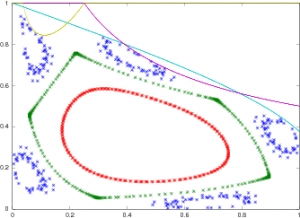# Archive | problems

## Topologically constrained models of statistical physics.

$$\def\Real{\mathbb{R}} \def\Int{\mathbb{Z}} \def\Comp{\mathbb{C}} \def\Rat{\mathbb{Q}} \def\Field{\mathbb{F}} \def\Fun{\mathbf{Fun}} \def\e{\mathbf{e}} \def\f{\mathbf{f}} \def\bv{\mathbf{v}} \def\blob{\mathcal{B}}$$

### The Blob

Consider the following planar “spin model”: the state of the system is a function from $$\Int^2$$ into $$\{0,1\}$$ (on and off states). We interpret the site $$(i,j), i,j\in\Int$$ as the plaque, i.e. the (closed) square given by the inequalities $$s_{ij}:=i-1/2\leq x\leq i+1/2; j-1/2\leq y\leq j+1/2$$.

To any configuration $$\eta$$ we associate the corresponding active domain,
$A_\eta=\bigcup_{(i,j): \eta(i,j)=1} s_{ij}.$

We are interested in the statistical ensembles supported by the finite contractible active domains – let’s refer to such domains as blobs.…

## hyperbolic geometry of Google maps

$$\def\Real{\mathbb{R}} \def\views{\mathbb{G}} \def\earth{\mathbb{E}} \def\hsp{\mathbb{H}}$$

##### Google Maps and the Space of Views

Let’s consider the geometry hidden behind one of the best user interfaces in mobile apps ever, – the smartphone maps, the ones which you can swipe, flick and pinch. We are not talking here about the incredible engine that generates the maps on the fly as your finger waddles over the screen – just about the interface, which in a very intuitive and remarkably efficient way allows you to move between various maps.…

## unimodal category

We say that a continuous function is unimodal if all the upper excursion sets
$$e_f(c):=\{f≥c\}$$ are contractible. For a nonnegative function
$$f:\mathbb{R}^d\to\mathbb{R}_+$$ with compact support its unimodal category UCat(f) as the least number $$u$$ of summands in a decomposition $$f=\sum_{i=1}^u f_i$$, where all $$f_i$$ are unimodal. Unimodal category is a “soft” version of Lyusternik-Schnirelman category, hence the name. We know how to compute UCat only in one-dimensional situation. In other dimensions, even the simplest questions seem hard, for example:

Find the unimodal category of the suspension of univariate Morse functions, i.e.

## linear searchLinear search problems deals with locating an object hidden on a half line (“long road”) by an absent-minded robot which gather everything it sees (see, e.g. here for more details). A program for the robot is a sequence $$0<x_1<x_2<\ldots$$ of excursions it takes until the object is found.

In the situation when the object is hidden at a random location with a known distribution function $$F$$, the problem of finding an optimal trajectory can be translated into understanding the dynamics of certain area preserving mappings.…